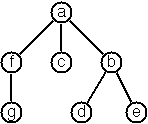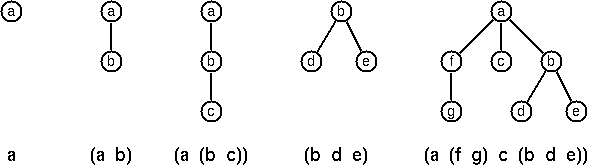# 99 questions/70B to 73

(Redirected from 99 questions/71 to 80)

This is part of Ninety-Nine Haskell Problems, based on Ninety-Nine Prolog Problems.

## Multiway Trees

A multiway tree is composed of a root element and a (possibly empty) set of successors which are multiway trees themselves. A multiway tree is never empty. The set of successor trees is sometimes called a forest.## Problem 70B

(*) Check whether a given term represents a multiway tree. (no solution needed)

In Prolog or Lisp, one writes a predicate to check this.

Example in Prolog:

```?- istree(t(a,[t(f,[t(g,[])]),t(c,[]),t(b,[t(d,[]),t(e,[])])])).
Yes
```

In Haskell, we define multiway trees as a datatype, as in the module Data.Tree:

```data Tree a = Node a [Tree a]
deriving (Eq, Show)
```

Some example trees:

```tree1 = Node 'a' []

tree2 = Node 'a' [Node 'b' []]

tree3 = Node 'a' [Node 'b' [Node 'c' []]]

tree4 = Node 'b' [Node 'd' [], Node 'e' []]

tree5 = Node 'a' [
Node 'f' [Node 'g' []],
Node 'c' [],
Node 'b' [Node 'd' [], Node 'e' []]
]
```

The last is the tree illustrated above.

As in Problem 54A, all members of this type are multiway trees; there is no use for a predicate to test them.

## Problem 70C

(*) Count the nodes of a multiway tree. Solutions

```λ> nnodes tree2
2
```

## Problem 70

(**) Construct a multiway tree from a node string. Solutions

We suppose that the nodes of a multiway tree contain single characters. In the depth-first order sequence of its nodes, a special character ^ has been inserted whenever, during the tree traversal, the move is a backtrack to the previous level.

By this rule, the tree below (tree5) is represented as: afg^^c^bd^e^^^Define the syntax of the string and write a predicate tree(String,Tree) to construct the Tree when the String is given. Make your predicate work in both directions.

```λ> stringToTree "afg^^c^bd^e^^^"
Node 'a' [Node 'f' [Node 'g' []],Node 'c' [],Node 'b' [Node 'd' [],Node 'e' []]]

λ> treeToString (Node 'a' [Node 'f' [Node 'g' []],Node 'c' [],Node 'b' [Node 'd' [],Node 'e' []]])
"afg^^c^bd^e^^^"
```

## Problem 71

(*) Determine internal path length of multiway tree. Solutions

We define the internal path length of a multiway tree as the total sum of the path lengths from the root to all nodes of the tree. By this definition, tree5 has an internal path length of 9.

```λ> ipl tree5
9
λ> ipl tree4
2
```

## Problem 72

(*) Construct bottom-up order sequence of multiway tree nodes. Solutions

Write a predicate bottom_up(Tree,Seq) which constructs the bottom-up sequence of the nodes of the multiway tree Tree.

```λ> bottom_up tree5
"gfcdeba"
```

## Problem 73

(**) Lisp-like multiway tree representation. Solutions

There is a particular notation for multiway trees in Lisp. Lisp is a prominent functional programming language, which is used primarily for artificial intelligence problems. As such it is one of the main competitors of Prolog. In Lisp almost everything is a list, just as in Prolog everything is a term.

The following pictures show how multiway tree structures are represented in Lisp.Note that in the "lispy" notation a node with successors (children) in the tree is always the first element in a list, followed by its children. The "lispy" representation of a multiway tree is a sequence of atoms and parentheses '(' and ')', which we shall collectively call "tokens". We can represent this sequence of tokens as a Prolog list; e.g. the lispy expression (a (b c)) could be represented as the Prolog list ['(', a, '(', b, c, ')', ')']. Write a predicate tree_ltl(T,LTL) which constructs the "lispy token list" LTL if the tree is given as term T in the usual Prolog notation.

(The Prolog example given is incorrect.)

```λ> display lisp tree1
"a"
λ> display lisp tree2
"(a b)"
λ> display lisp tree3
"(a (b c))"
λ> display lisp tree4
"(b d e)"
λ> display lisp tree5
"(a (f g) c (b d e))"
```

As a second, even more interesting exercise try to rewrite tree_ltl/2 in a way that the inverse conversion is also possible.